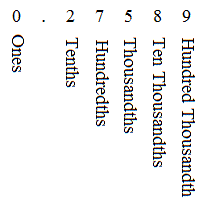Converting Decimals to Fractions: Part A

If a decimal number terminates (in other words, the digits don't go on forever), express the decimal number as a common fraction with a power of ten as the denominator.  Then reduce the fraction to lowest terms .

Since we need to put our number over a power of ten, we need to know what the various places after the decimal point mean.Example:

Express $0.425$ as a fraction in lowest terms.

Since there are $3$ digits in $425$ , the very last digit is the $1000$ th place.

So, $0.425$ is read $425$ thousandths.

It is written $\frac{425}{1000}$ . It is now in fraction form but not reduced to lowest terms.

$425$ and $1000$ have a common factor of $25$ , so we have to divide both the numerator and the denominator by $25$ to reduce the fraction.

$\frac{425}{1000}÷\frac{25}{25}=\frac{17}{40}$ .   Now the fraction is in lowest terms.